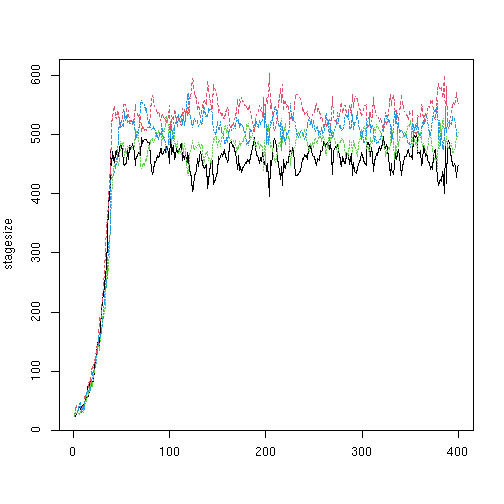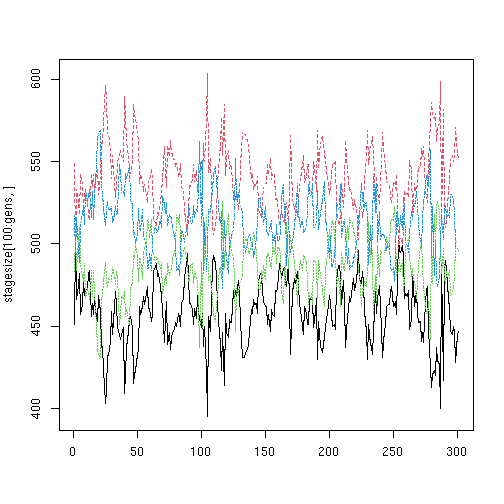When a population in rmetasim reaches carrying capacity, individuals are killed at random. That is, the probability of an individual dying during this thinning process is (N-K)/N where N is the size of a population and K is carrying capacity for that population.

There may be situations where one might want to apply the carrying capacity thinning with more delicacy. This vignette provides one such approach.

The idea is to break apart the components of a discrete time simulation implemented in the function 'landscape.simulate()' Rmetasim provides a full set of these components (see the vignette on setting up simulations).

In each time-click, landscape.simulate():

1. applies the extinction vector to the landscape
2. lets individuals reproduce
3. applies growth and survival
4. carrying capacity thinning is implemented

Each of these steps have been implemented in rmetasim functions (respectively):

1. landscape.extinct()
2. landscape.reproduce()
3. landscape.survive()
4. landscape.carry()

landscape.carry() can be replaced with a different approach to carrying capacity thinning..

###Setup landscape This will be a 2 habitat with 2 stages per habitat landscape

``````library(rmetasim)
``````
``````## Registered S3 method overwritten by 'spdep':
##   method   from
##   plot.mst ape
``````
``````## Registered S3 method overwritten by 'pegas':
##   method      from
``````
``````l <- landscape.new.example()
``````

###landscape.stagecarry()

This function will take a landscape and a matrix of stage proportions. If a population is above its carrying capacity, individuals will be removed based on these stage proportions. This matrix will have the number of rows equal to the number of habitats and the number of columns equal to the stages in a habitat.

Each cell in a matrix row will equal the proportion of individuals in that stage that should be thinned. In the following, the matrix is called SK. Each row should sum to 1. If they do not, they will be normalized before thinning.

``````##       stage 1 stage 2
## pop 1     0.8     0.2
## pop 2     0.5     0.5
``````

This matrix specifies that in pop1 80% of the individuals thinned for carrying capacity come from stage 1. Inb pop2 the individuals come from each of the stages equally. If there are not enough individuals in a stage to meet the carrying capacity limit by thinning, additional thinning will be applied at random to all stages in the population.

This function implements the stage carrying

``````landscape.stagecarry <- function(l,sk)
{
k=l\$demography\$epochs[]\$Carry # carrying capacities
n=c(table(landscape.populations(l))) #pop sizes

#next several lines are supposed to padd out extirpated populations in the popsize vector
n2=rep(0,length(k))
names(n2)=1:length(k)
for(i in names(n)) {pos=which(names(n2)==i); n2[pos]=n[names(n)==i]}
n=n2
killrows <- unlist(lapply(1:length(k),function(i) #never that many populations, this is probably plenty fast
{
if (n[i]>k[i]) #population i has a pop size greater than carrying capacity
{
poprows = which(landscape.populations(l)%in%i)
abstages = (i-1)*dim(sk) + 0:(dim(sk)-1)
absrows = lapply(abstages,function(stg)
{which(l\$individuals[,1]==stg)})
inds.to.thin = ceiling(sk[i,] * (n[i]-k[i]))
(sapply(1:dim(sk), function(stg) { if (length(absrows[[stg]])>0) sample(absrows[[stg]],inds.to.thin[stg],replace=F) }))
}
}))

if (!is.null(killrows)) l\$individuals<-l\$individuals[(-1)*killrows,]
l
}
``````

###Running the simulation

``````rland <- landscape.new.example()
rland\$demography\$localdem[]\$LocalS <- matrix(c(0.5,0.5,0,0.05),nrow=2) #make sure that there is some complexity
gens=400
stagesize=matrix(0,ncol=4,nrow=gens)
for (gen in 1:gens)
{

rland <- landscape.extinct(rland)
rland <- landscape.reproduce(rland)
rland <- landscape.survive(rland)
rland <- landscape.stagecarry(rland,SK)
stagesize[gen,]  <- c(table(rland\$individuals[,1]))

rland <- landscape.carry(rland)   #if N is still > K which happens if there are few inds in a stage that you want to thin
# apply the normal carry function (thins the remaining stages using the normal approach)
}
``````

These plots show the relative sizes of the different stages in the simulation. black and red are the stages in the first population and green and blue, population 2.

``````matplot(stagesize,type='l')
````````````matplot(stagesize[100:gens,],type="l") #clip the first 100 time clicks.  resets the index to zero for gen 100
``````